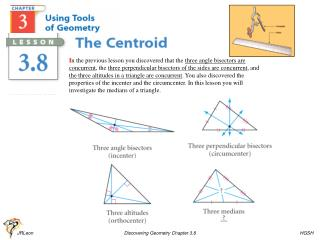DownloadDownload PresentationPg. 185

# Pg. 185

Download Presentation## Pg. 185

- - - - - - - - - - - - - - - - - - - - - - - - - - - E N D - - - - - - - - - - - - - - - - - - - - - - - - - - -
##### Presentation Transcript

1. In the previous lesson you discovered that the three angle bisectors are concurrent, the three perpendicular bisectors of the sides are concurrent, and the three altitudes in a triangle are concurrent. You also discovered the properties of the incenter and the circumcenter. In this lesson you will investigate the medians of a triangle. Pg. 185 JRLeon Discovering Geometry Chapter 3.8 HGSH

2. The point of concurrency of the three medians is the centroid. JRLeon Discovering Geometry Chapter 3.8 HGSH

3. http://mathopenref.com/trianglecentroid.html JRLeon Discovering Geometry Chapter 3.8 HGSH

4. In earlier lessons you discovered that the midpoint of a segment is the balance point or center of gravity. You also saw that when a set of segments is arranged into a triangle, the line through each midpoint of a side and the opposite vertex can act as a line of balance for the triangle. Can you then balance a triangle on a median? Let’s take a look. JRLeon Discovering Geometry Chapter 3.8 HGSH

5. The two triangles formed by the median have the same area. yes, the centroid If you have found the balancing point for the triangle, you have found its center of gravity. State your discovery as a conjecture, and add it to your conjecture list. JRLeon Discovering Geometry Chapter 3.8 HGSH

6. You have discovered special properties of three of the four points of concurrency—the incenter, the circumcenter, and the centroid. The incenter is the center of an inscribed circle, the circumcenter is the center of a circumscribed circle, and the centroid is the center of gravity. JRLeon Discovering Geometry Chapter 3.8 HGSH

7. Classwork / Homework Assignment: 3.8: p 188 problems 1 thru 5 (5 problems) JRLeon Discovering Geometry Chapter 3.8 HGSH

8. JRLeon Discovering Geometry Chapter 3.8 HGSH

9. JRLeon Discovering Geometry Chapter 3.8 HGSH

10. JRLeon Discovering Geometry Chapter 3.8 HGSH

11. JRLeon Discovering Geometry Chapter 3.8 HGSH

12. JRLeon Discovering Geometry Chapter 3.8 HGSH